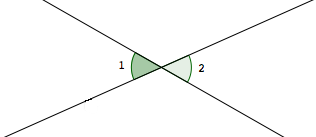﻿ Angles: Complementary, Supplementary, Vertical, and Linear Pairs

#Angles: Complementary, Supplementary, Vertical, and Linear Pairs

You may want to review:

For this web exercise, assume all angles are measured in degrees.

DEFINITIONS complementary angles, supplementary angles

Two angles are complementary  if and only if  the sum of their measures is $\,90^{\circ}\,.$

Two angles are supplementary  if and only if  the sum of their measures is $\,180^{\circ}\,.$

In particular:

• if $\,\angle 1\,$ and $\,\angle 2\,$ are complementary, then $\,m\angle 1 + m\angle 2 = 90^{\circ}\,$
• if $\,\angle 1\,$ and $\,\angle 2\,$ are supplementary, then $\,m\angle 1 + m\angle 2 = 180^{\circ}\,$
DEFINITION opposite rays

Rays that:

• share a common endpoint, and
• point in opposite directions
are called opposite rays.Note:  If three points are on a line with $\,B\,$ between $\,A\,$ and $\,C\,,$ then $\,\overrightarrow{BA}\,$ and $\,\overrightarrow{BC}\,$ are opposite rays.

Recall that both $\,A{-}B{-}C\,$ and $\,C{-}B{-}A\,$ are notation for ‘$B\,$ is between $\,A\,$ and $\,C\,$’.

DEFINITION linear pair

Two angles are a linear pair if and only if

• they have a common side, and
• their other sides are opposite rays.Note:  If $\,\angle 1\,$ and $\,\angle 2\,$ are a linear pair, then $\,m\angle 1 + m\angle 2 = 180^{\circ}\,.$

DEFINITION vertical angles

Two angles are vertical angles if and only if the sides of one angle are opposite rays to the sides of the other.Note:   Vertical angles are the ‘opposite angles’ that are formed by two intersecting lines.

Note:  If $\,\angle 1\,$ and $\,\angle 2\,$ are vertical angles, then $\,m\angle 1 = m\angle 2\ .$

DEFINITION parallel lines

Two lines are parallel if and only if they lie in the same plane and do not intersect.

Parallel lines are studied in more detail in a future section, Parallel Lines.

The symbol ‘$\,\parallel\,$’ is used to denote parallel lines.

The sentence ‘$\,\ell\parallel m\,$’ is read as ‘$\,\ell \,$ is parallel to $\,m\,$’, and is true precisely when line $\,\ell\,$ is parallel to line $\,m\,.$

DEFINITION perpendicular lines
Two lines are perpendicular if and only if they form a right angle.

The symbol ‘$\,\perp\,$’ is used to denote perpendicular lines.

The sentence ‘$\,\ell\perp m\,$’ is read as ‘$\,\ell \,$ is perpendicular to $\,m\,$’, and is true precisely when line $\,\ell\,$ is perpendicular to line $\,m\,.$

## Example

Question: Suppose that $\,\angle 1\,$ and $\,\angle 2\,$ are vertical angles. Suppose that  $\,m\angle 1 = 7x-15\,$   and  $\,m\angle 2 = 2x+55\,.$ Find $\,x\,.$
Solution:
 $7x-15= 2x+55$ vertical angles have equal measures $5x-15=55$ subtract $\,2x\,$ from both sides $5x=70$ add $\,15\,$ to both sides $x=14$ divide both sides by $\,5$